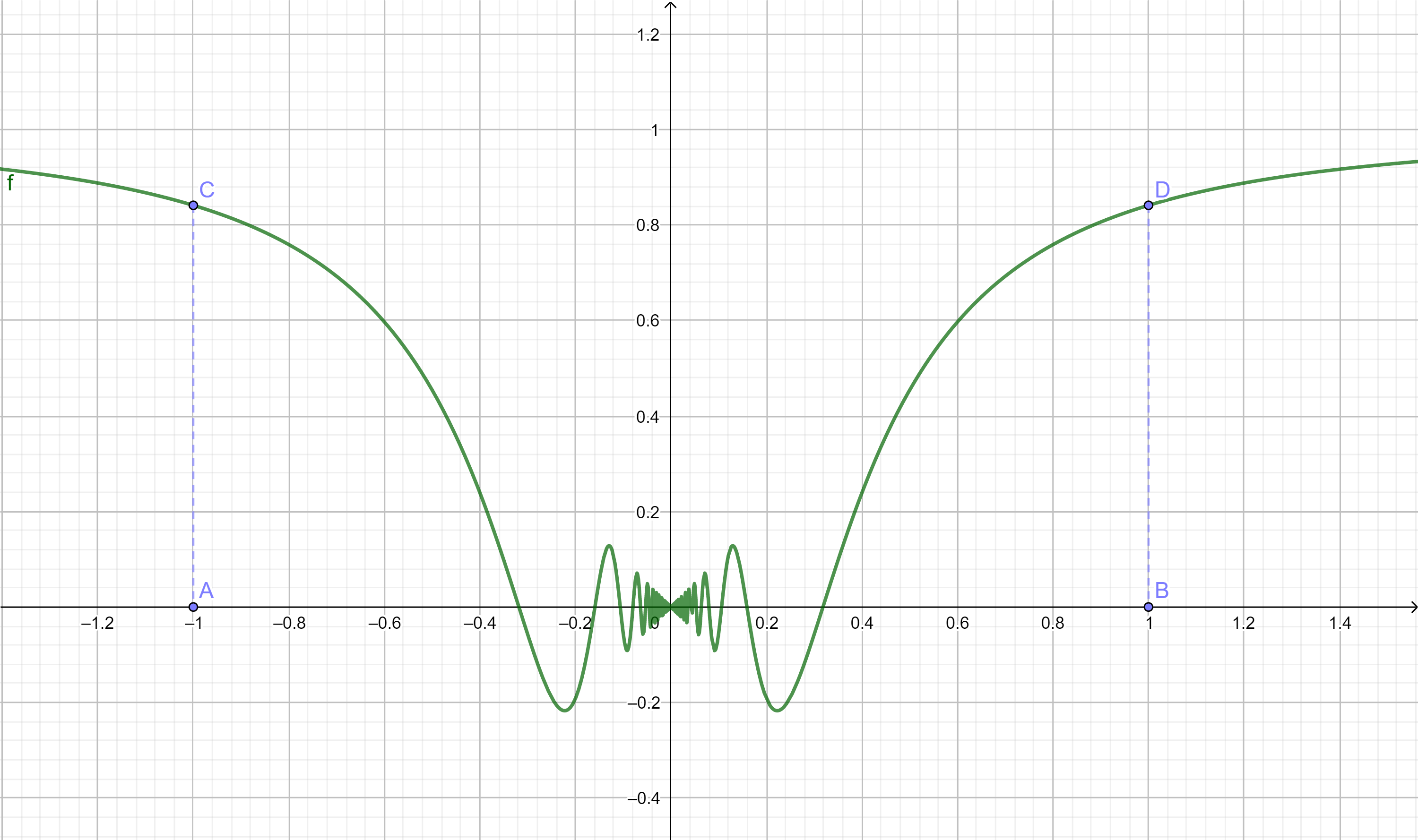Questions in category: 曲线积分 (Curvilinear Integral)

## 1. 求曲线的弧长

Posted by haifeng on 2021-10-14 09:30:04 last update 2021-10-14 09:52:31 | Answers (0) | 收藏

$f(x)=\begin{cases} x\sin\frac{1}{x}, & x\neq 0, x\in[-1,1]\\ 0, & x=0, \end{cases}$[Hint]

$\text{len}=2\int_{0}^{1}\sqrt{1+(f'(x))^2}\mathrm{d}x$

Rem. 这里的曲线在0点附近无限振荡, 直觉其长度为无穷, 但是否真的是无穷是要通过计算的. 如果是无穷, 请给出计算以证明.

## 2. 求星形线 $x^{2/3}+y^{2/3}=a^{2/3}$ 的弧长.

Posted by haifeng on 2015-01-25 19:49:47 last update 2015-01-25 19:52:34 | Answers (0) | 收藏

Hint:

$\begin{cases} x=a\cos^3\theta,\\ y=a\sin^3\theta, \end{cases}$

## 3. 利用曲线积分的知识证明球面上连接两点的可微曲线以过这两点的大圆劣弧的长度最短.

Posted by haifeng on 2014-09-10 16:54:02 last update 2014-09-10 16:54:02 | Answers (0) | 收藏

## 4. 计算摆线上的曲线积分

Posted by haifeng on 2014-08-30 20:42:37 last update 2014-08-31 16:32:19 | Answers (1) | 收藏

$\int_{L}\cos(x+y^2)dx+\biggl[2y\cos(x+y^2)-\frac{1}{\sqrt{1+y^4}}\biggr]dy,$

$\begin{cases} &x=a(t-\sin t),\\ &y=a(1-\cos t), \end{cases}$